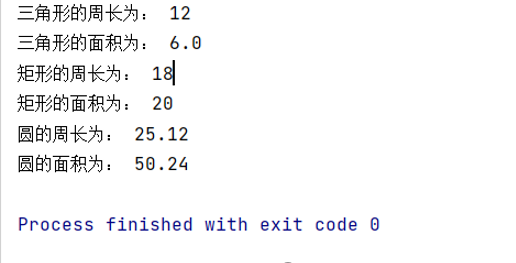• java定义一个抽象类shape
千次阅读
2021-03-15 18:18:44

定义一个抽象类Shape，成员有图形名称(name)和求面积的抽象方法Area(),利用继承产生子类三角形类Trangle类,圆Circle类,矩形Rectangle类。并分别实现计算面积的方法计算相应图形的面积。对于Trangle类要求能够实现修改三边，判断三边是否能够构成三角形，根据三边长计算面积的方法。

abstract class shape

{

String name;

abstract void Area();

}

class Trangle extends shape //三角形类

{ Trangle(){}

double sideA,sideB,sideC;

boolean boo;

public Trangle(double a,double b,double c)

{

sideA=a;sideB=b;sideC=c;

this.name="三角形";

if(a+b>c&&a+c>b&&b+c>a)

{

System.out.println("我是一个三角形");

boo=true;

}

else

{

System.out.println("我不是一个三角形");

boo=false;

}

}

public void Area()

{

if(boo)

{

double p=(sideA+sideB+sideC)/2.0;

double area=Math.sqrt(p*(p-sideA)*(p-sideB)*(p-sideC));

System.out.println(name+"面积是:"+area);

}

else

{

System.out.println("不是一个三角形，不能计算面积");

}

}

public void 修改三边(double a,double b,double c)

{

sideA=a;sideB=b;sideC=c;

if(a+b>c&&a+c>b&&b+c>a)

{

boo=true;

}

else

{

boo=false;

}

}

}

class Circle extends shape //圆类

{

double r;

Circle(double r)

{

this.r=r;this.name="圆";

}

public void Area()

{

System.out.println(name+"面积是:"+3.14*r*r);

}

}

class Rectangle extends shape //矩形类

{

double a,b;

Rectangle(double a,double b)

{

this.a=a;this.b=b;this.name="矩形";

}

public void Area()

{

System.out.println(name+"面积是:"+a*b);

}

}

class 图形1

{

public static void main(String[] args)

{

Trangle t=new Trangle(1,2,3);

t.Area();

t.修改三边(3,4,5);

t.Area();

Circle c=new Circle(2);

c.Area();

Rectangle r=new Rectangle(4,5);

r.Area();

}

}

对于上题目中的Shape类，改用接口来实现同样的功能

interface shape

{

final String name="图形";

void Area();

}

class Trangle implements shape

{

Trangle(){}

double sideA,sideB,sideC;

boolean boo;

public Trangle(double a,double b,double c)

{

sideA=a;sideB=b;sideC=c;

if(a+b>c&&a+c>b&&b+c>a)

{

System.out.println("我是一个三角形");

boo=true;

}

else

{

System.out.println("我不是一个三角形");

boo=false;

}

}

public void Area()

{

if(boo)

{

double p=(sideA+sideB+sideC)/2.0;

double area=Math.sqrt(p*(p-sideA)*(p-sideB)*(p-sideC));

System.out.println(name+"面积:"+area);

}

else

{

System.out.println("不是一个三角形，不能计算面积");

}

}

public void 修改三边(double a,double b,double c)

{

sideA=a;sideB=b;sideC=c;

if(a+b>c&&a+c>b&&b+c>a)

{

boo=true;

}

else

{

boo=false;

}

}

}

class Circle implements shape //圆类

{

double r;

Circle(double r)

{

this.r=r;

}

public void Area()

{

System.out.println(name+"面积是:"+3.14*r*r);

}

}

class Rectangle implements shape //矩形类

{

double a,b;

Rectangle(double a,double b)

{

this.a=a;this.b=b;

}

public void Area()

{

System.out.println(name+"面积是:"+a*b);

}

}

class A

{

public void t(shape s) //接口类型参数

{

s.Area(); //接口回调

}

}

class 图形2

{

public static void main(String[] args)

{

shape s;

s=new Trangle(3,4,5);

s.Area() ; //接口回调

A a=new A();

a.t(new Circle(2));

a.t(new Rectangle(3,4));

}

}

更多相关内容
• 使用抽象类定义一个“形状类”，能够计算周长和面积，分别用矩形、三角形、圆形对形状类进行继承，并定义测试类进行测试输出周长和面积。
• 定义一个抽象类Shape（有两个纯虚函数）派生出Circle和Rectangle，并实现相应的纯虚函数，再定义一个类CAR，使用CAR来统一调用Circle和Rectangle
• 定义一个抽象类shape,它包含一个抽象方法getArea() 定义一个抽象类Shape,它包含一个抽象方法getArea()，从Shape类派生出Rectangle和Circle类，这两个类都用getArea()方法计算对象面积，编写应用程序使用Rectangle...

## 定义一个抽象类shape,它包含一个抽象方法getArea()

定义一个抽象类Shape,它包含一个抽象方法getArea()，从Shape类派生出Rectangle和Circle类，这两个类都用getArea()方法计算对象面积，编写应用程序使用Rectangle和Circle类。

实验目的：定义Rectangle和Circle类的构造方法，初始化成员变量，并计算出面积。

代码如下（示例）：

package com.ljc.Abstract;

//创建了Shape抽象类
abstract class Shape {

//写抽象方法getArea
abstract double getArea();
}

//继承Shape抽象类派生出Rectangle类
class Rectangle extends Shape {
double a, b;

//构造方法
Rectangle() {
}
//初始化变量
Rectangle(double a, double b) {
this.a = a;
this.b = b;
}
//输入方法
double getA(double a) {
this.a = a;
return a;
}
//输入方法
double getB(double b) {
this.b = b;
return b;
}
//输出方法，抽象方法调用
double getArea() {
return (a * b) / 2;
}
}

//解释同上
class Circle extends Shape {
double r;

Circle() {
}

Circle(double r) {
this.r = r;
}

double getR(double r) {
this.r=r;
return r;
}
//使用调用Java库使用math方法，把Π的值调出来
double getArea() {
return r*r*Math.PI;
}
}


测试方法:
新建一个ShapeTest类
自行优化测试代码提示
package com.ljc.Abstract;

public class ShapeTest {

public static void main(String[] args) {

//测试方法就不过多介绍
Rectangle a=new Rectangle();
a.getA(60.4);
a.getB(35.1);
System.out.println(a.getArea());
Circle b=new Circle();
b.getR(3);
System.out.println(b.getArea());
}

}


展开全文抽象类 java
• 1) 编写一个抽象类Shape，其中有抽象方法getArea（）和getPerimeter（） 2) 在Shape类的基础上派生出Rectangle和Circle类，二者都实现了计算面积的方法getArea（）和计算周长的方法getPerimeter（）； 3) 构造main...抽象类Shape

* (程序头部注释开始)

* 程序的版权和版本声明部分

* 文件名称：定义抽象类shape和由它派生出来的三个派生类。

* 作    者：         晁阳

* 完成日期：     2012    年 05      月  15  日

* 版 本 号：       t1.0

* 对任务及求解方法的描述部分

* 输入描述： 定义抽象类shape和由它派生出来的三个派生类。

* 问题描述：

* 程序输出：用如下的mian()函数，求出定义的几个几何体的面积和。

* 程序头部的注释结束

*/

#include

using namespace std;

class Shape

{

public:

virtual double area() = 0;

};

class Circle:public Shape

{

public:

Circle(double a):a(a){};

virtual double area();

private:

double a;

};

class Rectangle:public Shape

{

public:

Rectangle(double b,double c):b(b),c(c){};

virtual double area();

private:

double b;

double c;

};

class Triangle:public Shape

{

public:

Triangle(double d,double e):d(d),e(e){};

virtual double area();

private:

double d;

double e;

};

double Circle::area( )

{

return (3.14159*a*a);

}

double Rectangle::area()

{

return ( b*c);

}

double Triangle::area()

{

return ( e*d/2 );

}

int main()

{

Circle c1(12.6),c2(4.9); //建立Circle类对象c1,c2,参数为圆半径

Rectangle r1(4.5,8.4),r2(5.0,2.5); //建立Rectangle类对象r1,r2,参数为矩形长、宽

Triangle t1(4.5,8.4),t2(3.4,2.8); //建立Triangle类对象t1,t2，参数为三角形底边长与高

Shape *pt={&c1,&c2,&r1,&r2,&t1,&t2}; //定义基类指针数组pt，各元素指向一个派生类对象

double areas=0.0; //areas为总面积

for(int i=0; i<6; i++)

{

areas=areas+pt[i]->area();

}

cout<

system("pause");

return 0;

}

上图：展开全文• class Shape: def __init__(self,areas=0,primeters=0): self.areas=areas self.Primeters=primeters def GetPrimeter(self): pass def GetArea(self): pass class Triangle(Shape): def __init__(self,said...
class Shape:
def __init__(self,areas=0,primeters=0):
self.areas=areas
self.Primeters=primeters
def GetPrimeter(self):
pass
def GetArea(self):
pass
class Triangle(Shape):
def __init__(self,said1=0,said2=0,said3=0,areas=0,primeters=0):
super().__init__(areas,primeters)
self.said1=said1
self.said2=said2
self.said3=said3
def GetPrimeter(self):
self.Primeters=(self.said1+self.said2+self.said3)
def GetArea(self):
s = (self.said1 + self.said2 + self.said3) / 2
self.areas = ((s * (s - self.said1)) * (s - self.said2) * (s - self.said3))**0.5
class Rectangle(Shape):
def __init__(self,length=0,width=0,areas=0,primeters=0):
super().__init__(areas,primeters)
self.length=length
self.width=width
def GetPrimeter(self):
self.Primeters=(self.length+self.width)*2
def GetArea(self):
self.areas=self.length*self.width
class Circle(Shape):
def __init__(self,r=0,areas=0,primeters=0):
super().__init__(areas,primeters)
self.r=r
def GetPrimeter(self):
self.Primeters=2*3.14*self.r
def GetArea(self):
self.areas=3.14*self.r*self.r
a1=Triangle(3,4,5)
a1.GetPrimeter()
a1.GetArea()
print("三角形的周长为：",a1.Primeters)
print("三角形的面积为：",a1.areas)

a2=Rectangle(4,5)
a2.GetPrimeter()
a2.GetArea()
print("矩形的周长为：",a2.Primeters)
print("矩形的面积为：",a2.areas)

a3=Circle(4)
a3.GetPrimeter()
a3.GetArea()
print("圆的周长为：",a3.Primeters)
print("圆的面积为：",a3.areas)展开全文python
• Java定义抽象类Shape，其中包含抽象方法double getPeremeter( )求周长和double getArea( )求面积。 定义一个矩形类，继承此抽象类，并自行扩充成员变量和方法，定义一个方法一次显示长和宽、周长和面积。java
• 我之前做了简易计算器的简单工厂模式,效果与你这是一样的~~~~你的抽象类Shape里面的方法area()和perimeter(),可以参考的计算器的GetResult()都是虚方法(使用virtual 关键字),然后在计算面积、周长的子类中分别...
• 定义一个抽象类Shpae表示形状，给定颜色属性String color,提供一个不带参数的构造方法和一个带参数的构造方法，完成对属性的赋值，提供一个计算面积的抽象方法area()。定义一个类Circle表示圆形，继承于Shape，给定...java 算法
• （1）定义一个抽象类Shape，在该类中： 创建抽象方法Area()和printArea()， Area() 求解并返回面积，printArea() 用于输出面积，无返回值。 （2）创建Shape类的子类Rectangle（矩形）类，该类中： 包括两个私有...java
• abstract class Shape{ //声明一个返回float类型的抽象方法area() //声明一个没有返回值的抽象方法printArea() } class Rectangle extends Shape{ int width; int length; public Rectangle(int newWidth,int ...java
• 定义一个抽象类Shape，该类中包含两个成员变量x,y,用来记录图形的中心位置，同时在该类中还包含一个非抽象方法void ShowCenter()和一个抽象方法double Area()，分别用来显示图形的中心位置和求图形的面积，从该抽象...java
• 展开全部没啥大问题，既然返回是e68a8462616964757a686964616f31333365656530double那么输入就用double还有记住每一个类一个java文件，不要放在一个java文件中！！！Shape.java类publicabstractclassShape{...
• Test测试代码： package com.tengxun.Animal; public class ShapeTest { public static void main(String[] args) { //实例化圆和矩形 Circle c1=new Circle(4);//圆的半径自己可以设 Rectangle r1=new ...java
• 编写一个抽象类Shape，声明计算图形面积的抽象方法。再分别定义Shape的子类Circle（圆）和Rectangle（矩形），在两个子类中按照不同图形的面积计算公式，实现Shape类中计算面积的方法。定义测试类ShapeTest对圆和...java
• class Shape //定义抽象基类 Shape{public:virtual double area() const =0; //纯虚函数};class Circle:public Shape{public:Circle(double r):radius(r){} //构造函数virtual double area() const /...
• 定义一个抽象类shape,它包含一个抽象方法getArea(),从Shape类派生除Rectangle和Circle类，这两个类都用getArea()方法计算对象的面积。编写应用程序使用Rectangle和Circle类。 代码如下： abstract class Shape { ...抽象类
• 可能的Java代码： import java.util.*; abstract class Shape { public abstract Double calculateShape(); } class Rectangle extends Shape { private double length; private double width; public ...
• 定义一个抽象类shape ，用来表示一般图形。shape具有属性name，表示图形的名字，如三角形、矩形、圆形，抽象方法area和perimeter，分别用来计算形状的面积和周长。（1）定义一个Rectangle类继承shape类，定义矩形的...java
• 、实验内容： 1．学生类和借书卡类的设计 要求：（1）设计学生类、借书卡类、及应用测试类3类； （2）在学生类中设计属性：学生名字和...2．定义抽象类Shape（形状）其中有抽象方法用来求某形状的周长和面积；定义java 面向对象编程 继承 抽象类
• 设计一个抽象类图形类，在该类中包含有至少两个抽象方法求周长和求面积，分别定义圆形类、长方形类、正方形类、三角形类来继承图形类，并实现上述两个方法 设计抽象类 就要使用abstract关键字，抽象类中的抽象方法要...
• JAVA实现一个类层次：Shape、Rectangle、Triangle，其中：Shape类定义一个抽象方法area().mip版关注:281答案:3悬赏:50解决时间 2021-01-26 21:52已解决2021-01-26 01:40用JAVA实现一个类层次：Shape、...
• C+编程：编写一个程序,定义抽象基类Shape，由它派生出5个派生：Cricle(圆形),Square(正方形)， Rectangle(矩形)， Trapezoid (梯形),Triangle(三角形)。用虚函数分别计算图形面积，并求所有图形面积的和．要求用...
• 抽象类,用来表示一个抽象概念. 是一种只能定义类型,而不能产生对象的类,所以定义抽象类则必须有子类的出现. 抽象类的好处在于能够明确地定义子类需要覆写的方法 抽象类需要使用abstract声明. 抽象类里可以实现抽象...抽象类 设计模式 java 多态 接口
• Shape.javapackage project2.second;public abstract class Shape {public abstract double perimetre(double length,double width,double radius);public abstract double area(double length,double width,double ...
• 请编写一个抽象类Shape，在此基础上派生出类Rectangle和Circle，二者都有计算对象面积的函数getArea()、计算对象周长的函数getPerim() #include &amp;lt;iostream&amp;gt; #include &amp;lt;...C++ 抽象类 继承
• 定义抽象类Shapes，其中包含抽象方法：计算面积getArea(),计算 周长getPerimeter()。 定义矩形类rectangle、圆类Crcle都由抽象类Shapes派生而来，定义相应的属性，构造方法，并实现getArea ()igetPerimeter()抽象...java 面向对象编程 抽象类 继承...